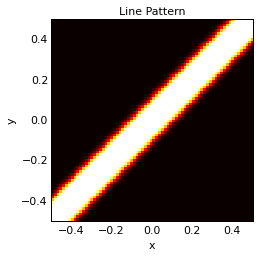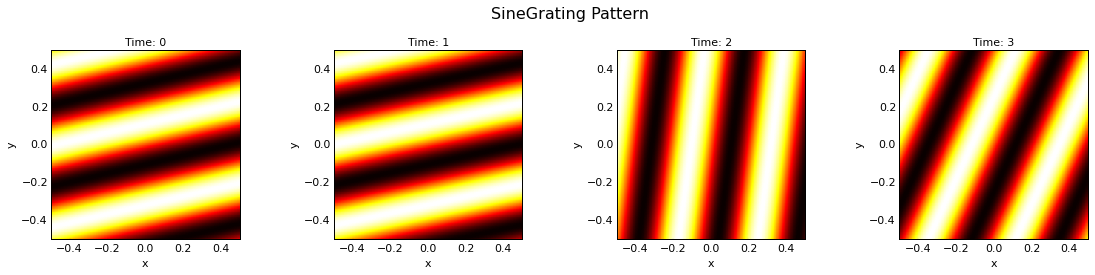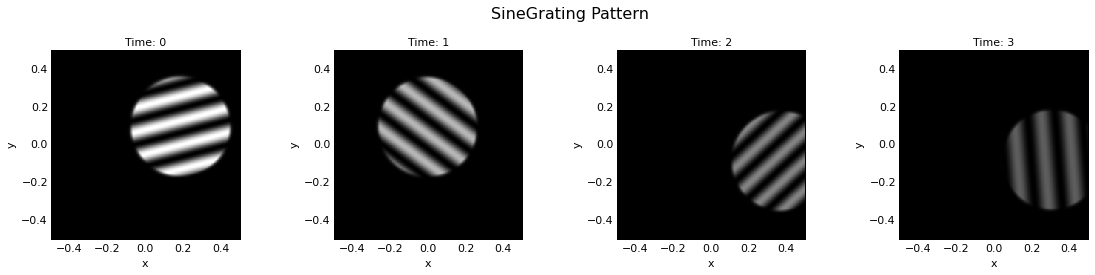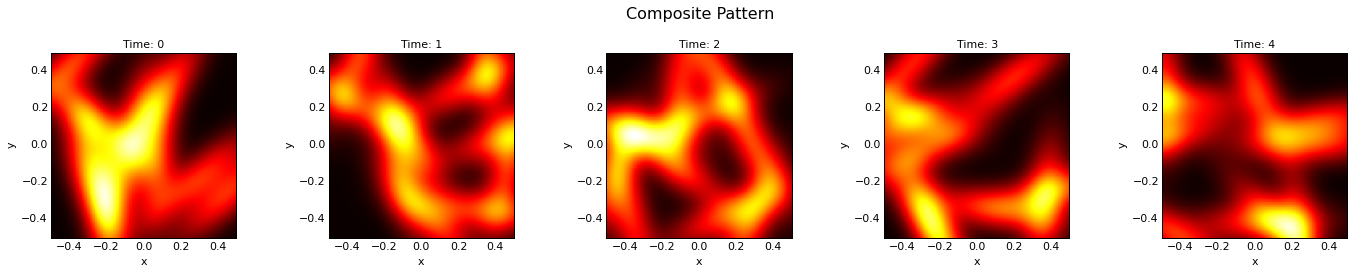# ImaGen¶The ImaGen package provides comprehensive support for creating resolution-independent one- and two-dimensional pattern distributions. ImaGen consists of a large library of primarily two-dimensional spatial patterns, including mathematical functions, geometric primitives, and images read from files, along with many ways to combine or select from any other patterns. These patterns can be used in any Python program that needs configurable patterns or streams of patterns. Basically, as long as the code can accept a Python callable and will call it each time it needs a new pattern, users can then specify any pattern possible in ImaGen's simple declarative pattern language, and the downstream code need not worry about any of the details about how the pattern is specified or generated. This approach gives users full flexibility about which patterns they wish to use, while relieving the downstream code from having to implement anything about patterns. The detailed examples below should help make this clear.

## Usage¶

To create a pattern, just import imagen, then instantiate one of ImaGen's PatternGenerator classes. Each of these classes support various parameters, which are each described in the Reference Manual or via help(pattern-object-or-class). Any parameter values specified on instantiation become the defaults for that object:

In :
import imagen as ig
line=ig.Line(xdensity=5, ydensity=5, smoothing=0)


Then whenever the line object is called, you'll get a new NumPy array:

In :
line()

Out:
array([[ 0.,  0.,  0.,  0.,  0.],
[ 0.,  0.,  0.,  0.,  0.],
[ 1.,  1.,  1.,  1.,  1.],
[-0., -0., -0., -0., -0.],
[-0., -0., -0., -0., -0.]])

Here the parameters xdensity and ydensity specified that a continuous 1.0×1.0 region in (x,y) space should be sampled on a 5×5 grid. The line object can now be called repeatedly, with any parameter values specified to override those declared above:

In :
import numpy as np
np.set_printoptions(1)
line(smoothing=0.1,orientation=0.8,thickness=0.4)

Out:
array([[ 0. ,  0.1,  0.7,  1. ,  1. ],
[ 0.1,  0.7,  1. ,  1. ,  1. ],
[ 0.7,  1. ,  1. ,  1. ,  0.7],
[ 1. ,  1. ,  1. ,  0.7,  0.1],
[ 1. ,  1. ,  0.7,  0.1,  0. ]])

ImaGen depends only on NumPy, Param, and HoloViews, none of which have any other required dependencies, and it is thus easy to incorporate ImaGen into your own code to generate or use patterns freely. An optional interface to matplotlib via HoloViews is also available, which provides a convenient way to plot the pattern objects:

In :
import holoviews

line.set_param(xdensity=72,ydensity=72,orientation=np.pi/4, thickness=0.1, smoothing=0.02)
line[:]

The holoviews.ipython extension is already loaded. To reload it, use:

Out:We will use this plotting interface to show off the remaining patterns, but please remember that the main purpose of ImaGen is to generate arrays for use in other programs, not simply to draw pretty patterns for plotting!

### Dynamic parameter values¶

As you can see above, PatternGenerator objects return different patterns depending on their parameter values. An important feature of these parameter values is that any of them can be set to "dynamic" values, which will then result in a different pattern each time (see the Param package and its numbergen module for details). With dynamic parameters, PatternGenerators provide streams of patterns, not just individual patterns. For example, let's define a SineGrating object with a random orientation, collect four of them at different times (using the .anim() method), and lay them out next to each other (using the NdLayout class from HoloViews):

In :
import numbergen as ng
from holoviews import NdLayout
import param
param.Dynamic.time_dependent=True
NdLayout(ig.SineGrating(orientation=np.pi*ng.UniformRandom()).anim(3))

Out:As you can see, each time the sine grating was rendered, the pattern differed, because the parameter value for orientation was chosen randomly. Of course, you can set any combination of patterns to dynamic values, to get arbitrarily complex variation over time:

In :
%%opts Image (cmap='gray')
sine_disk = ig.SineGrating(orientation=np.pi*ng.UniformRandom(),
scale=0.25*ng.ExponentialDecay(time_constant=3),
frequency=4+7*ng.UniformRandom(),
x=0.3*ng.NormalRandom(seed=1),
y=0.2*ng.UniformRandom(seed=2)-0.1,
NdLayout(sine_disk.anim(3))

Out:### Composite patterns¶

As you can see above, PatternGenerator objects can also be used as a mask for another PatternGenerator, which is one simple way to combine them.

PatternGenerators can also be combined directly with each other to create Composite PatternGenerators, which can make any possible 2D pattern. For instance, we can easily sum 10 oriented Gaussian patterns, each with random positions and orientations, giving a different overall pattern at each time:

In :
gs = ig.Composite(operator=np.add,
generators=[ig.Gaussian(size=0.15,
x=ng.UniformRandom(seed=i+1)-0.5,
y=ng.UniformRandom(seed=i+2)-0.5,
orientation=np.pi*ng.UniformRandom(seed=i+3))
for i in range(10)])

NdLayout(gs.anim(4)).cols(5)

Out:Once it has been defined, a Composite pattern works just like any other pattern, so that it can be placed, rotated, combined with others, etc., allowing you to build up arbitrarily complex objects out of simple primitives. Here we created a Composite pattern explicitly, but it's usually easier to create them by simply using any of the usual Python operators (+, -, *, /, **, %, & (min), and | (max)) as in the examples below.

For instance, here's an example using np.maximum (via the | operator on PatternGenerators), rotating the composite pattern together as a unit. We also leave it as a HoloViews animation rather than laying it out over space:

In :
%%opts Image.Pattern (cmap='Blues_r')
l1 = ig.Line(orientation=-np.pi/4)
l2 = ig.Line(orientation=+np.pi/4)
cross = l1 | l2
cross.orientation=ng.ScaledTime()*(np.pi/-20)
l1.anim(20) + l2.anim(20) + cross.anim(20)

Out: